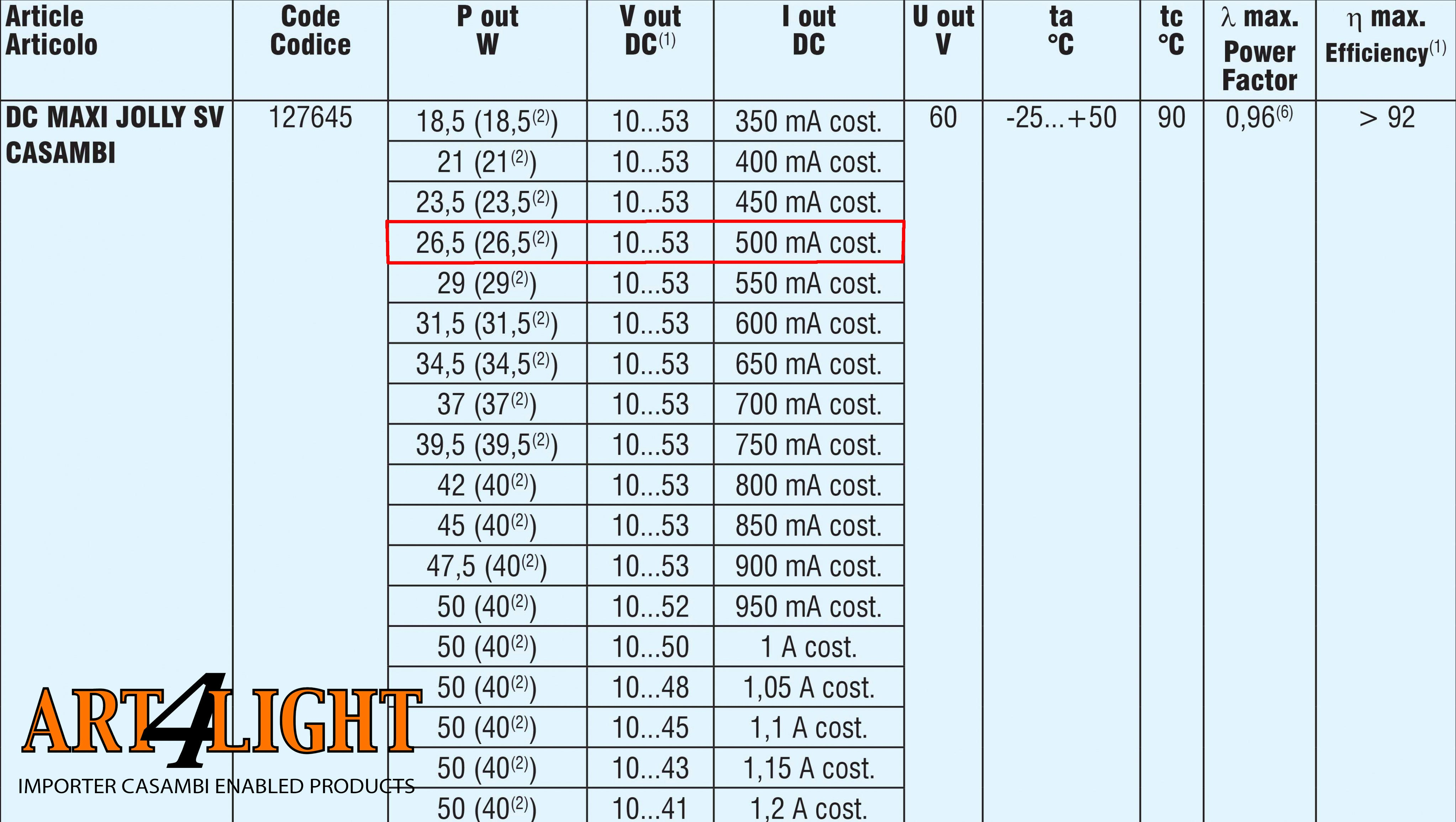Casambi drivers that feed your LEDs with a mA circuit (milliamps) will always try to maintain the current strength.

Example;

You have two 500mA LEDs in series, each with a power of 10W.
So joint power is 20W.

In the table below you can see that a TCI Maxijolly SV could be sufficient for you. In the red frame you first go to '500mA' in the third column, which is your LED current value. Afterwards go to the left and you will see that the driver output can vary between a minimum of 10V and a maximum of 53V. One more column to the left you can see that the maximum connected power is 26,5W.First a little bit of teaching about a law;
P = U x I either
POWER = VOLTAGE X CURRENT either
Watt = Volt x Ampere

After you put 230V on it, the driver will increase the voltage in an instant until it reaches the current of 500mA. In my example, this means that the voltage will be at the output of the driver; U = P / I or U = 20W / 0,5A (500mA) = 40Volt.

Because the LEDs are in series, you measure a voltage of 20V over each LED.

------

Suppose you use long wires from the driver to the LEDs, a voltage drop will occur along the length of the wire. It is based on a misunderstanding that this voltage loss causes the LEDs to give less light. The driver keeps the number of mA that it 'sends' and will simply increase the voltage at the output slightly. The driver can do this until it has reached its maximum voltage. Casambi drivers that accept long wires include TCI and ELDOled.

First a little bit of teaching about a law;
U = I x R either
VOLTAGE = CURRENT x RESISTANCE either
Volt = Ampere x Ohm.

Now suppose you are going to put a 20 meter copper wire between the driver and the LEDs and that wire has a resistance of, for example, 4 ohms? That 500mA also goes through that long wire! What does this mean for your LEDs and the light output? Nothing! watch;

U = I x R either
U = 0,5 (500mA) x 4 ohms = 2Volt.

To maintain that current of 500mA, the driver will simply increase the voltage to; 40 + 2 = 42 Volt, where the LEDs do not even know that the long wire is in between.

Finally, I would like to inform you that strictly speaking the power (P) in the above examples should not be expressed in Watts but in VA. In this blog you can put that aside for a moment, but in another blog where I write about the difference between actual and apparent flows and capacities, it will be explained.• Irma Lankhaar